## The quantum mechanical view of the atom

8-10-99

### Heisenberg uncertainty principle

The uncertainty principle is a rather interesting idea, stating that it is not possible to measure both the position and momentum of a particle with infinite precision. It also states that the more accurately you measure a particle's position, the less accurately you're able to measure it's momentum, and vice versa.

This idea is really not relevant when you're making measurements of large objects. It is relevant, however, when you're looking at very small objects such as electrons. Consider that you're trying to measure the position of an electron. To do so, you bounce photons off the electron; by figuring out the time it takes for each photon to come back to you, you can figure out where the electron is. The more photons you use, the more precisely you can measure the electron's position.

However, each time a photon bounces off the electron, momentum is transferred to the electron. The more photons you use, the more momentum is transferred, and because you can't measure that momentum transferred to infinite precision the more uncertainty you're introducing in the measurement of the momentum of the electron.

Heisenberg showed that there is a limit to the accuracy you can measure things: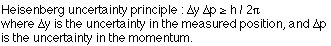The uncertainty can also be stated in terms of the energy of a particle in a particular state, and the time in which the particle is in that state: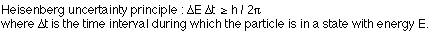### Quantum numbers

The Bohr model of the atom involves a single quantum number, the integer n that appears in the expression for the energy of an electron in an orbit. This picture of electrons orbiting a nucleus in well-defined orbits, the way planets orbit the Sun, is not our modern view of the atom. We now picture the nucleus surrounded by electron clouds, so the orbitals are not at all well-defined; we still find the Bohr theory to be useful, however, because it gives the right answer for the energy of the electron orbitals.

The Bohr model uses one quantum number, but a full quantum mechanical treatment requires four quantum numbers to characterize the electron orbitals. These are known as the principal quantum number, the orbital quantum number, the magnetic quantum number, and the spin quantum number. These are all associated with particular physical properties.

n, the principal quantum number, is associated with the total energy, the same way it is in the Bohr model. In fact, calculating the energy from the quantum mechanical wave function gives the expression Bohr derived for the energy: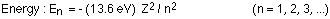, the orbital quantum number, is connected to the total angular momentum of the electron. This quantum number is an integer less than n, and the total angular momentum of the electron can be calculated using: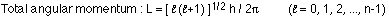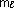, the magnetic quantum number, is related to one particular component of the angular momentum. By convention, we call this the z-component. The energy of any orbital depends on the magnetic quantum number only when the atom is in an external magnetic field. This quantum number is also an integer; it can be positive or negative, but it has a magnitude less than or equal to the orbital quantum number. The z-component of the electron's angular momentum is given by: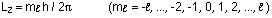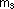, the spin quantum number is related to something called the spin angular momentum of the electron. The closest analogy is that it's similar to the Earth spinning on its axis. There are only two possible states for this quantum number, often referred to as spin up and spin down.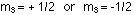What's the use of having all these quantum numbers? We need all four to completely describe the state an electron occupies in the atom.

### Electron probability density clouds

A very important difference between the Bohr model and the full quantum mechanical treatment of the atom is that Bohr proposed that the electrons were found in very well-defined circular orbits around the nucleus, while the quantum mechanical picture of the atom has the electron essentially spread out into a cloud. We call this a probability density cloud, because the density of the cloud tells us what the probability is of finding the electron at a particular distance from the nucleus.

In quantum mechanics, something called a wave function is associated with each electron state in an atom. The probability of finding an electron at a particular distance from the nucleus is related to the square of the wave function, so these electron probability density clouds are basically three-dimensional pictures of the square of the wave function.

### The Pauli exclusion principle

If you've got a hydrogen atom, with only a single electron, it's very easy to determine the possible states that electron can occupy. A particular state means one particular combination of the 4 quantum numbers; there are an infinite number of states available, but the electron is more likely to occupy a low-energy state (i.e., a low n state) than a higher-energy (higher n) state.

What happens for other elements, when there is more than one electron to worry about? Can all the electrons be found in one state, the ground state, for example? It turns out that this is forbidden: the Pauli exclusion principle states that no two electrons can occupy the same state. In other words, no two electrons can have the same set of 4 quantum numbers.

### Shells and subshells

As usual, for historical reasons we have more than one way to characterize an electron state in an atom. We can do it using the 4 quantum numbers, or we can use the notion of shells and subshells. A shell consists of all those states with the same value of n, the principal quantum number. A subshell groups all the states within one shell with the same value of, the orbital quantum number.

The subshells are usually referred to by letters, rather than by the corresponding value of the orbital quantum number. The letters s, p, d, f, g, and h stand for values of 0, 1, 2, 3, 4, and 5, respectively. Using these letters allows us to use a shorthand to denote how many electrons are in a subshell; this is useful for specifying the ground state (lowest energy state) of a particular atom.

The ground state configuration for oxygen, for instance, can be written as :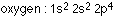This means that the lowest energy configuration of oxygen, with 8 electrons, is to have two electrons in the n=1 s-subshell, two in the n=2 s-subshell, and four in the n=2 p-subshell.

Potassium (Z = 19) has an interesting ground state configuration: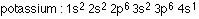That's interesting because there is a d-subshell in the n = 3 shell, but instead of the last electron going into that subshell it goes into the s-subshell of the n=4 shell. It does this to minimize the energy: the 4s subshell is at a lower energy than the 3d subshell.

### The periodic table

When Mendeleev organized the elements into the periodic table, he knew nothing about quantum numbers and subshells. The way the elements are organized in the periodic table, however, is directly related to how the electrons fill the levels in the different shells.

Different columns of the periodic table group elements with similar properties; they have similar properties because of the similarities between their ground state electron configurations. The noble gases (He, Ne, Ar, etc.) are all in the right-most column of the periodic table. Their ground state configurations have no partially filled subshells; having a complete subshell is favorable from the standpoint of minimizing energy so these elements do not react readily.

On the other hand, the column next to the noble gases is the halogens; these are one electron short of having completely-filled subshells, so if they can share an electron from another element they're happy to do so. They react readily with elements whose ground state configurations have a single electron in one subshell like the alkali metals (Li, Na, K, etc.).

WebElements is a nice web-based periodic table, having all sorts of useful information about different elements. Click on the icon below to check it out.